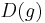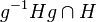# Randomized black-box group algorithm for normality testing has false positive rate of at most three-fourths

## Statement

Suppose$G$ is a finite group and$H$ is a subgroup of$G$ that is not normal in$G$. In other words, there exist$g \in G$ and$h \in H$ such that$ghg^{-1} \notin H$. Then, suppose$C$ is the set:$C = \{(g,h) \mid g \in G, h \in H, ghg^{-1} \in H \}$

Then we have:$\! |C| \le \frac{3}{4} |G||H|$

In other words, the probability that a randomly selected pair from$G \times H$ is in$C$ is at most$3/4$.

## Related facts

### Applications

The fact has applications for the normality testing problem, and forms the basis of the randomized black-box group algorithm for normality testing.

## Facts used

1. Lagrange's theorem in the special form subgroup of size more than half is whole group

## Proof

Given: A finite group$G$, subgroup$H$ that is not normal in$G$.$C = \{(g,h) \mid g \in G, h \in H, ghg^{-1} \in H \}$

To prove:$|C| \le \frac{3}{4}|G||H|$

Proof:

Step no. Assertion/construction Facts used Given data used Previous steps used Explanation
1 Let$A$ be the set of elements in$G$ that do not normalize$H$. Then,$|A| \ge \frac{1}{2}|G|$. Fact (1)$H$ is not normal in$G$,$G$ is finite The normalizer$N_G(H)$ is a subgroup of$G$. Since$H$ is not normal, it is a proper subgroup of$G$. Hence, by Fact (1), it has size at most half the size of$G$. Therefore, its complement,$A$, has size at least half the size of$G$.
2 For$g \in A$, let$D(g)$ be the set of elements$h \in H$ such that$ghg^{-1} \notin H$. Then,$|D(g)| \ge \frac{1}{2}|H|$. Fact (1) Step (1) Since$g$ does not normalize$H$, we have that$g^{-1}Hg$ is not contained in$H$. Thus,$g^{-1}Hg \cap H$ is a proper subgroup of$H$. By Fact (1), it has size at most half the size of$H$. Therefore, its complement in$H$, which is$D(g)$, has size at least half the size of$H$.
3$\sum_{g \in A} |D(g)| \ge \frac{1}{4} |G||H|$ Steps (1), (2) Direct combination of steps
4$|C| \le \frac{3}{4}|G||H|$ Steps (1), (2), (3) The complement of$C$ in$G \times H$ is precisely the set of pairs$(g,h)$ where$g \in A$ and$h \in D(g)$. By Step (3), the number of such pairs is at least$\frac{1}{4}|G||H|$, so the size of the complement is at most$\frac{3}{4}|G||H|$.